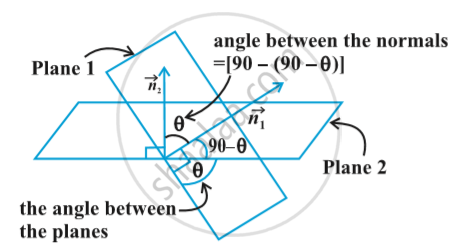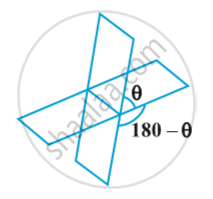# Angle Between Two Planes

#### definition

The angle between two planes is defined as the angle between their normals Fig,#### notes

Observe that if θ is an angle between the two planes, then so is 180 – θ Fig.If vec n _1 and vec n_2 are normals to the planes and θ be the angle between the planes
vec r . vec n _1 = d_1 and vec r . vec n _2 = d_2 .
Then θ is the angle between the normals to the planes drawn from some common point We have
cos θ = |(vec n_1 . vec n_2)/ (|vec n _1| |vec n_2|)|

Cartesian form
Let θ be the angle between the planes,
A_1x + B_1y +C_1z + D_1 = 0 and A_2x +B_2y + C_2 z + D_2 = 0
The direction ratios of the normal to the planes are A_1, B_1, C_1 and A_2, B_2, C_2 respectively.
Therefore , cos θ = |(A_1 A_2 + B_1 B_2 + C_1 C_2)/ (sqrt(A_1^2 + B_1^2 + C_1^2 ) sqrt (A_2^2 + B_2^2 +C_2^2))|

If you would like to contribute notes or other learning material, please submit them using the button below.

#### Video Tutorials

We have provided more than 1 series of video tutorials for some topics to help you get a better understanding of the topic.

Series 1

Series 2

### Shaalaa.com

Three Dimensional Geometry Part 6 - The Plane [00:34:04]
S
0%